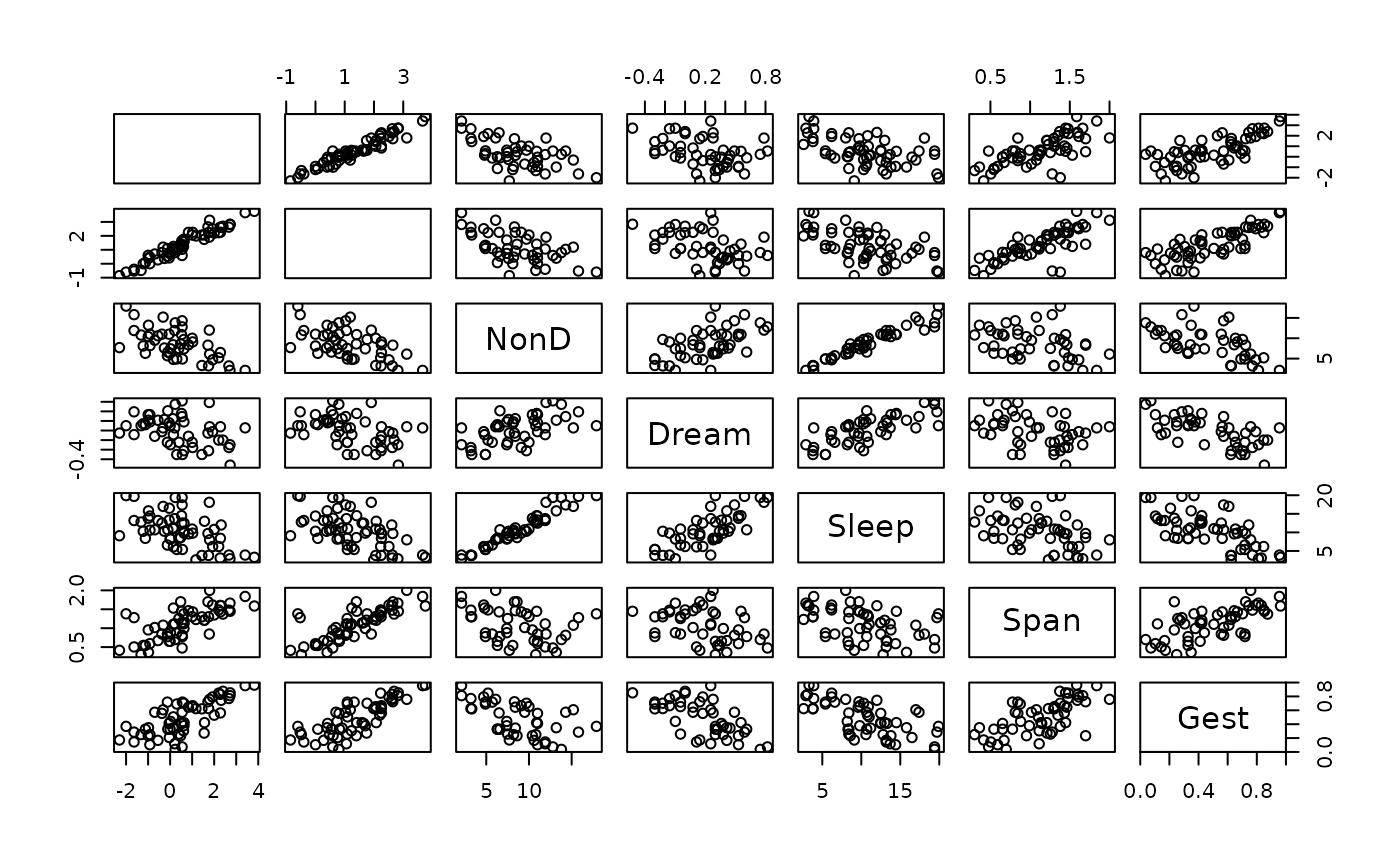Create a scatterplot matrix.

pairsVIM(
x,
...,
delimiter = NULL,
main = NULL,
sub = NULL,
panel = points,
lower = panel,
upper = panel,
diagonal = NULL,
labels = TRUE,
pos.labels = NULL,
cex.labels = NULL,
font.labels = par("font"),
layout = c("matrix", "graph"),
gap = 1
)

## Arguments

x

a matrix or data.frame.

...

further arguments and graphical parameters to be passed down. par("oma") will be set appropriately unless supplied (see graphics::par()).

delimiter

a character-vector to distinguish between variables and imputation-indices for imputed variables (therefore, x needs to have colnames()). If given, it is used to determine the corresponding imputation-index for any imputed variable (a logical-vector indicating which values of the variable have been imputed). If such imputation-indices are found, they are used for highlighting and the colors are adjusted according to the given colors for imputed variables (see col).

main, sub

main and sub title.

panel

a function(x, y, ...{}), which is used to plot the contents of each off-diagonal panel of the display.

lower, upper

separate panel functions to be used below and above the diagonal, respectively.

diagonal

optional function(x, ...{}) to be applied on the diagonal panels.

labels

either a logical indicating whether labels should be plotted in the diagonal panels, or a character vector giving the labels.

pos.labels

the vertical position of the labels in the diagonal panels.

cex.labels

the character expansion factor to be used for the labels.

font.labels

the font to be used for the labels.

layout

a character string giving the layout of the scatterplot matrix. Possible values are "matrix" (a matrix-like layout with the first row on top) and "graph" (a graph-like layout with the first row at the bottom).

gap

a numeric value giving the distance between the panels in margin lines.

## Details

This function is the workhorse for marginmatrix() and scattmatrixMiss().

The graphical parameter oma will be set unless supplied as an argument.

A panel function should not attempt to start a new plot, since the coordinate system for each panel is set up by pairsVIM.

## Note

The code is based on graphics::pairs(). Starting with version 1.4, infinite values are no longer removed before passing the x and y vectors to the panel functions.

marginmatrix(), scattmatrixMiss()

Other plotting functions: aggr(), barMiss(), histMiss(), marginmatrix(), marginplot(), matrixplot(), mosaicMiss(), parcoordMiss(), pbox(), scattJitt(), scattMiss(), scattmatrixMiss(), spineMiss()

## Author

Andreas Alfons, modifications by Bernd Prantner

## Examples


data(sleep, package = "VIM")
x <- sleep[, -(8:10)]
x[,c(1,2,4,6,7)] <- log10(x[,c(1,2,4,6,7)])
pairsVIM(x)
#> Warning: variable 'Dream' contains infinite values